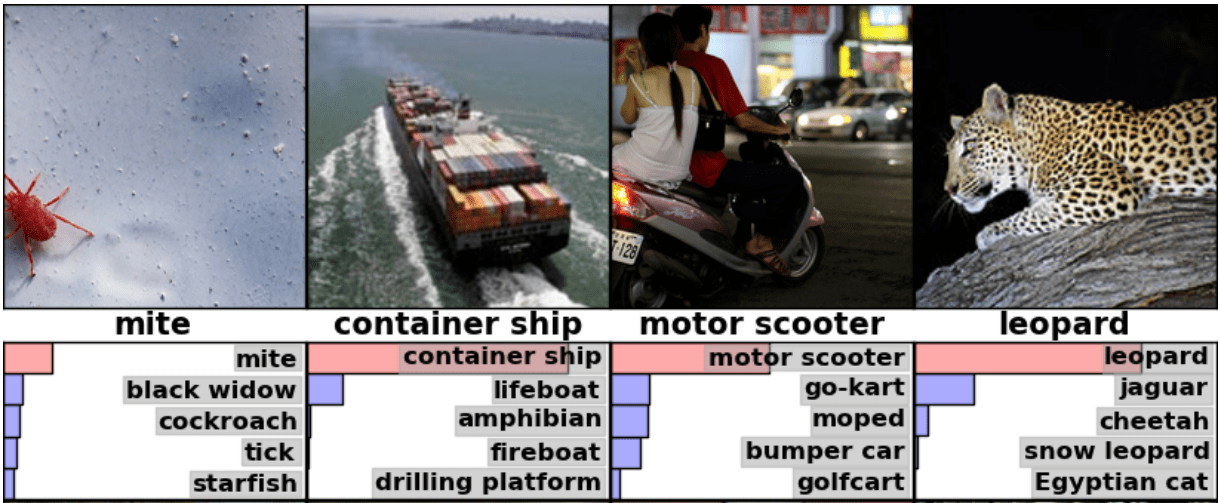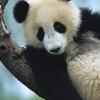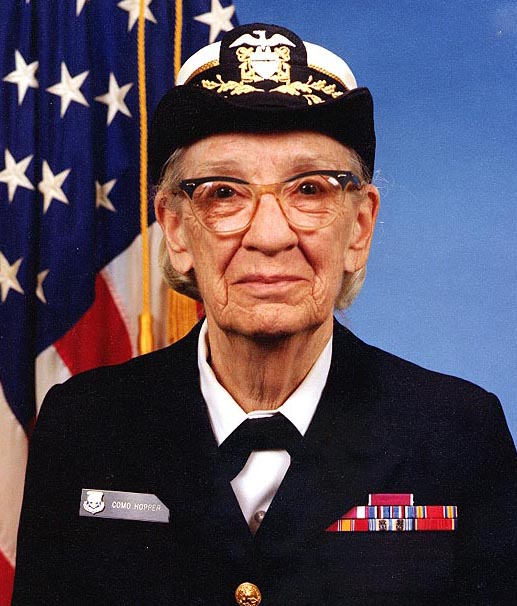# W3cubDocs

/TensorFlow Guide

# Image Recognition

Our brains make vision seem easy. It doesn't take any effort for humans to tell apart a lion and a jaguar, read a sign, or recognize a human's face. But these are actually hard problems to solve with a computer: they only seem easy because our brains are incredibly good at understanding images.

In the last few years, the field of machine learning has made tremendous progress on addressing these difficult problems. In particular, we've found that a kind of model called a deep convolutional neural network can achieve reasonable performance on hard visual recognition tasks -- matching or exceeding human performance in some domains.

Researchers have demonstrated steady progress in computer vision by validating their work against ImageNet -- an academic benchmark for computer vision. Successive models continue to show improvements, each time achieving a new state-of-the-art result: QuocNet, AlexNet, Inception (GoogLeNet), BN-Inception-v2. Researchers both internal and external to Google have published papers describing all these models but the results are still hard to reproduce. We're now taking the next step by releasing code for running image recognition on our latest model, Inception-v3.

Inception-v3 is trained for the ImageNet Large Visual Recognition Challenge using the data from 2012. This is a standard task in computer vision, where models try to classify entire images into 1000 classes, like "Zebra", "Dalmatian", and "Dishwasher". For example, here are the results from AlexNet classifying some images:To compare models, we examine how often the model fails to predict the correct answer as one of their top 5 guesses -- termed "top-5 error rate". AlexNet achieved by setting a top-5 error rate of 15.3% on the 2012 validation data set; Inception (GoogLeNet) achieved 6.67%; BN-Inception-v2 achieved 4.9%; Inception-v3 reaches 3.46%.

How well do humans do on ImageNet Challenge? There's a blog post by Andrej Karpathy who attempted to measure his own performance. He reached 5.1% top-5 error rate.

This tutorial will teach you how to use Inception-v3. You'll learn how to classify images into 1000 classes in Python or C++. We'll also discuss how to extract higher level features from this model which may be reused for other vision tasks.

We're excited to see what the community will do with this model.

## Usage with Python API

`classify_image.py` downloads the trained model from `tensorflow.org` when the program is run for the first time. You'll need about 200M of free space available on your hard disk.

Start by cloning the TensorFlow models repo from GitHub. Run the following commands:

```cd models/tutorials/image/imagenet
python classify_image.py
```

The above command will classify a supplied image of a panda bear.If the model runs correctly, the script will produce the following output:

```giant panda, panda, panda bear, coon bear, Ailuropoda melanoleuca (score = 0.88493)
indri, indris, Indri indri, Indri brevicaudatus (score = 0.00878)
lesser panda, red panda, panda, bear cat, cat bear, Ailurus fulgens (score = 0.00317)
custard apple (score = 0.00149)
earthstar (score = 0.00127)
```

If you wish to supply other JPEG images, you may do so by editing the `--image_file` argument.

If you download the model data to a different directory, you will need to point `--model_dir` to the directory used.

## Usage with the C++ API

You can run the same Inception-v3 model in C++ for use in production environments. You can download the archive containing the GraphDef that defines the model like this (running from the root directory of the TensorFlow repository):

```curl -L "https://storage.googleapis.com/download.tensorflow.org/models/inception_v3_2016_08_28_frozen.pb.tar.gz" |
tar -C tensorflow/examples/label_image/data -xz
```

Next, we need to compile the C++ binary that includes the code to load and run the graph. If you've followed the instructions to download the source installation of TensorFlow for your platform, you should be able to build the example by running this command from your shell terminal:

```bazel build tensorflow/examples/label_image/...
```

That should create a binary executable that you can then run like this:

```bazel-bin/tensorflow/examples/label_image/label_image
```

This uses the default example image that ships with the framework, and should output something similar to this:

```I tensorflow/examples/label_image/main.cc:206] military uniform (653): 0.834306
I tensorflow/examples/label_image/main.cc:206] mortarboard (668): 0.0218692
I tensorflow/examples/label_image/main.cc:206] academic gown (401): 0.0103579
I tensorflow/examples/label_image/main.cc:206] pickelhaube (716): 0.00800814
I tensorflow/examples/label_image/main.cc:206] bulletproof vest (466): 0.00535088
```

In this case, we're using the default image of Admiral Grace Hopper, and you can see the network correctly identifies she's wearing a military uniform, with a high score of 0.8.Next, try it out on your own images by supplying the --image= argument, e.g.

```bazel-bin/tensorflow/examples/label_image/label_image --image=my_image.png
```

If you look inside the `tensorflow/examples/label_image/main.cc` file, you can find out how it works. We hope this code will help you integrate TensorFlow into your own applications, so we will walk step by step through the main functions:

The command line flags control where the files are loaded from, and properties of the input images. The model expects to get square 299x299 RGB images, so those are the `input_width` and `input_height` flags. We also need to scale the pixel values from integers that are between 0 and 255 to the floating point values that the graph operates on. We control the scaling with the `input_mean` and `input_std` flags: we first subtract `input_mean` from each pixel value, then divide it by `input_std`.

These values probably look somewhat magical, but they are just defined by the original model author based on what he/she wanted to use as input images for training. If you have a graph that you've trained yourself, you'll just need to adjust the values to match whatever you used during your training process.

You can see how they're applied to an image in the `ReadTensorFromImageFile()` function.

```// Given an image file name, read in the data, try to decode it as an image,
// resize it to the requested size, and then scale the values as desired.
Status ReadTensorFromImageFile(string file_name, const int input_height,
const int input_width, const float input_mean,
const float input_std,
std::vector<Tensor>* out_tensors) {
tensorflow::GraphDefBuilder b;
```

We start by creating a `GraphDefBuilder`, which is an object we can use to specify a model to run or load.

```string input_name = "file_reader";
string output_name = "normalized";
b.opts().WithName(input_name));
```

We then start creating nodes for the small model we want to run to load, resize, and scale the pixel values to get the result the main model expects as its input. The first node we create is just a `Const` op that holds a tensor with the file name of the image we want to load. That's then passed as the first input to the `ReadFile` op. You might notice we're passing `b.opts()` as the last argument to all the op creation functions. The argument ensures that the node is added to the model definition held in the `GraphDefBuilder`. We also name the `ReadFile` operator by making the `WithName()` call to `b.opts()`. This gives a name to the node, which isn't strictly necessary since an automatic name will be assigned if you don't do this, but it does make debugging a bit easier.

```// Now try to figure out what kind of file it is and decode it.
const int wanted_channels = 3;
if (tensorflow::StringPiece(file_name).ends_with(".png")) {
} else {
// Assume if it's not a PNG then it must be a JPEG.
}
// Now cast the image data to float so we can do normal math on it.
tensorflow::Node* float_caster = tensorflow::ops::Cast(
// The convention for image ops in TensorFlow is that all images are expected
// to be in batches, so that they're four-dimensional arrays with indices of
// [batch, height, width, channel]. Because we only have a single image, we
// have to add a batch dimension of 1 to the start with ExpandDims().
tensorflow::Node* dims_expander = tensorflow::ops::ExpandDims(
float_caster, tensorflow::ops::Const(0, b.opts()), b.opts());
// Bilinearly resize the image to fit the required dimensions.
tensorflow::Node* resized = tensorflow::ops::ResizeBilinear(
dims_expander, tensorflow::ops::Const({input_height, input_width},
b.opts().WithName("size")),
b.opts());
// Subtract the mean and divide by the scale.
tensorflow::ops::Div(
tensorflow::ops::Sub(
resized, tensorflow::ops::Const({input_mean}, b.opts()), b.opts()),
tensorflow::ops::Const({input_std}, b.opts()),
b.opts().WithName(output_name));
```

We then keep adding more nodes, to decode the file data as an image, to cast the integers into floating point values, to resize it, and then finally to run the subtraction and division operations on the pixel values.

```// This runs the GraphDef network definition that we've just constructed, and
// returns the results in the output tensor.
tensorflow::GraphDef graph;
TF_RETURN_IF_ERROR(b.ToGraphDef(&graph));
```

At the end of this we have a model definition stored in the b variable, which we turn into a full graph definition with the `ToGraphDef()` function.

```std::unique_ptr<tensorflow::Session> session(
tensorflow::NewSession(tensorflow::SessionOptions()));
TF_RETURN_IF_ERROR(session->Create(graph));
TF_RETURN_IF_ERROR(session->Run({}, {output_name}, {}, out_tensors));
return Status::OK();
```

Then we create a `tf.Session` object, which is the interface to actually running the graph, and run it, specifying which node we want to get the output from, and where to put the output data.

This gives us a vector of `Tensor` objects, which in this case we know will only be a single object long. You can think of a `Tensor` as a multi-dimensional array in this context, and it holds a 299 pixel high, 299 pixel wide, 3 channel image as float values. If you have your own image-processing framework in your product already, you should be able to use that instead, as long as you apply the same transformations before you feed images into the main graph.

This is a simple example of creating a small TensorFlow graph dynamically in C++, but for the pre-trained Inception model we want to load a much larger definition from a file. You can see how we do that in the `LoadGraph()` function.

```// Reads a model graph definition from disk, and creates a session object you
// can use to run it.
std::unique_ptr<tensorflow::Session>* session) {
tensorflow::GraphDef graph_def;
return tensorflow::errors::NotFound("Failed to load compute graph at '",
graph_file_name, "'");
}
```

If you've looked through the image loading code, a lot of the terms should seem familiar. Rather than using a `GraphDefBuilder` to produce a `GraphDef` object, we load a protobuf file that directly contains the `GraphDef`.

```  session->reset(tensorflow::NewSession(tensorflow::SessionOptions()));
Status session_create_status = (*session)->Create(graph_def);
if (!session_create_status.ok()) {
return session_create_status;
}
return Status::OK();
}
```

Then we create a Session object from that `GraphDef` and pass it back to the caller so that they can run it at a later time.

The `GetTopLabels()` function is a lot like the image loading, except that in this case we want to take the results of running the main graph, and turn it into a sorted list of the highest-scoring labels. Just like the image loader, it creates a `GraphDefBuilder`, adds a couple of nodes to it, and then runs the short graph to get a pair of output tensors. In this case they represent the sorted scores and index positions of the highest results.

```// Analyzes the output of the Inception graph to retrieve the highest scores and
// their positions in the tensor, which correspond to categories.
Status GetTopLabels(const std::vector<Tensor>& outputs, int how_many_labels,
Tensor* indices, Tensor* scores) {
tensorflow::GraphDefBuilder b;
string output_name = "top_k";
tensorflow::ops::TopK(tensorflow::ops::Const(outputs, b.opts()),
how_many_labels, b.opts().WithName(output_name));
// This runs the GraphDef network definition that we've just constructed, and
// returns the results in the output tensors.
tensorflow::GraphDef graph;
TF_RETURN_IF_ERROR(b.ToGraphDef(&graph));
std::unique_ptr<tensorflow::Session> session(
tensorflow::NewSession(tensorflow::SessionOptions()));
TF_RETURN_IF_ERROR(session->Create(graph));
// The TopK node returns two outputs, the scores and their original indices,
// so we have to append :0 and :1 to specify them both.
std::vector<Tensor> out_tensors;
TF_RETURN_IF_ERROR(session->Run({}, {output_name + ":0", output_name + ":1"},
{}, &out_tensors));
*scores = out_tensors;
*indices = out_tensors;
return Status::OK();
```

The `PrintTopLabels()` function takes those sorted results, and prints them out in a friendly way. The `CheckTopLabel()` function is very similar, but just makes sure that the top label is the one we expect, for debugging purposes.

At the end, `main()` ties together all of these calls.

```int main(int argc, char* argv[]) {
// We need to call this to set up global state for TensorFlow.
tensorflow::port::InitMain(argv, &argc, &argv);
Status s = tensorflow::ParseCommandLineFlags(&argc, argv);
if (!s.ok()) {
LOG(ERROR) << "Error parsing command line flags: " << s.ToString();
return -1;
}

// First we load and initialize the model.
std::unique_ptr<tensorflow::Session> session;
string graph_path = tensorflow::io::JoinPath(FLAGS_root_dir, FLAGS_graph);
return -1;
}
```

We load the main graph.

```// Get the image from disk as a float array of numbers, resized and normalized
// to the specifications the main graph expects.
std::vector<Tensor> resized_tensors;
string image_path = tensorflow::io::JoinPath(FLAGS_root_dir, FLAGS_image);
image_path, FLAGS_input_height, FLAGS_input_width, FLAGS_input_mean,
FLAGS_input_std, &resized_tensors);
return -1;
}
const Tensor& resized_tensor = resized_tensors;
```

Load, resize, and process the input image.

```// Actually run the image through the model.
std::vector<Tensor> outputs;
Status run_status = session->Run({ {FLAGS_input_layer, resized_tensor}},
{FLAGS_output_layer}, {}, &outputs);
if (!run_status.ok()) {
LOG(ERROR) << "Running model failed: " << run_status;
return -1;
}
```

Here we run the loaded graph with the image as an input.

```// This is for automated testing to make sure we get the expected result with
// the default settings. We know that label 866 (military uniform) should be
// the top label for the Admiral Hopper image.
if (FLAGS_self_test) {
bool expected_matches;
Status check_status = CheckTopLabel(outputs, 866, &expected_matches);
if (!check_status.ok()) {
LOG(ERROR) << "Running check failed: " << check_status;
return -1;
}
if (!expected_matches) {
LOG(ERROR) << "Self-test failed!";
return -1;
}
}
```

For testing purposes we can check to make sure we get the output we expect here.

```// Do something interesting with the results we've generated.
Status print_status = PrintTopLabels(outputs, FLAGS_labels);
```

Finally we print the labels we found.

```if (!print_status.ok()) {
LOG(ERROR) << "Running print failed: " << print_status;
return -1;
}
```

The error handling here is using TensorFlow's `Status` object, which is very convenient because it lets you know whether any error has occurred with the `ok()` checker, and then can be printed out to give a readable error message.

In this case we are demonstrating object recognition, but you should be able to use very similar code on other models you've found or trained yourself, across all sorts of domains. We hope this small example gives you some ideas on how to use TensorFlow within your own products.

EXERCISE: Transfer learning is the idea that, if you know how to solve a task well, you should be able to transfer some of that understanding to solving related problems. One way to perform transfer learning is to remove the final classification layer of the network and extract the next-to-last layer of the CNN, in this case a 2048 dimensional vector. There's a guide to doing this in the how-to section.

## Resources for Learning More

To learn about neural networks in general, Michael Nielsen's free online book is an excellent resource. For convolutional neural networks in particular, Chris Olah has some nice blog posts, and Michael Nielsen's book has a great chapter covering them.

To find out more about implementing convolutional neural networks, you can jump to the TensorFlow deep convolutional networks tutorial, or start a bit more gently with our MNIST starter tutorial. Finally, if you want to get up to speed on research in this area, you can read the recent work of all the papers referenced in this tutorial.